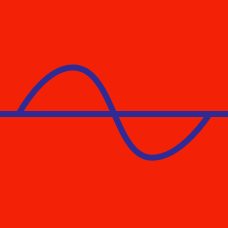Geometry

Graphs of Trigonometric Functions Warmup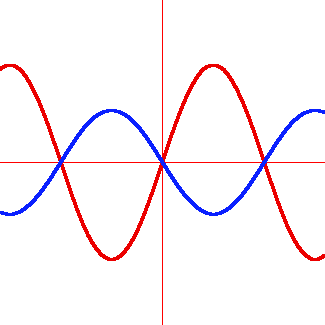If the graph in red is $y = 3\sin(x)$, what could the graph in blue be?

What's the minimum possible value of $y$ for

$y = -5 \sin(2x - \pi) - 2 ?$

The $\arccos$ function is typically defined by taking the inverse of a cosine with a domain of $[0, \pi)$. What would be the effect if the inverse was of a cosine with a domain of $[0, 2\pi)$ instead? (The graphs below may help.)

Graph of $f(x) = \cos(x)$: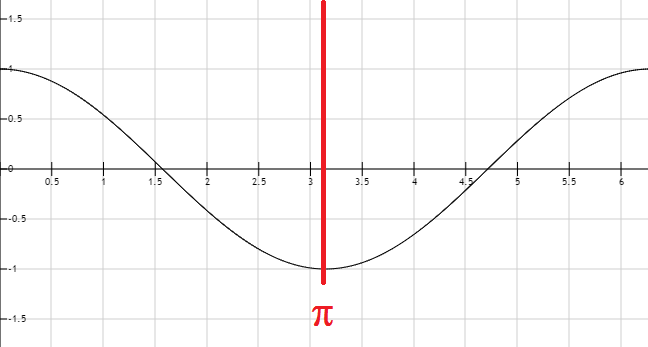Graph of $f(x) = \arccos(x)$: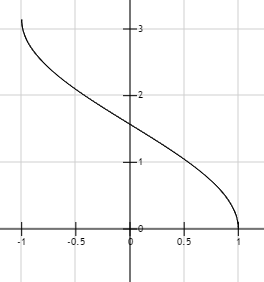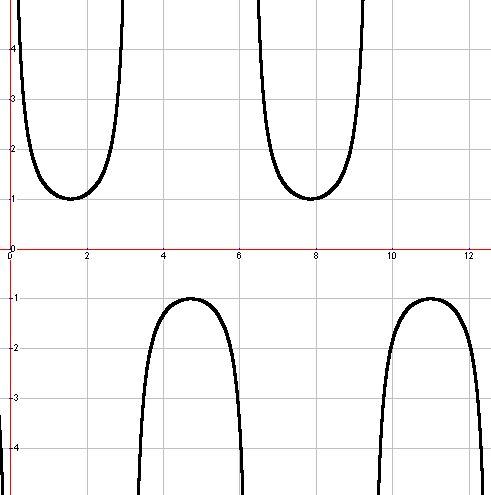The interval depicted is 0 to $4 \pi$. Which of these could not represent the graph above?

Starting from the basic definition of the tangent function, which is these facts most directly implies the tangent graph has a vertical asymptote at $\frac{\pi}{2}$?

×